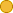## ← 08x-01 SEIR Model

• 1 Follower
• 42 Lines

### Get Embed Code x Embed video Use the following code to embed this video. See our usage guide for more details on embedding. Paste this in your document somewhere (closest to the closing body tag is preferable): ```<script type="text/javascript" src='https://amara.org/embedder-iframe'></script> ``` Paste this inside your HTML body, where you want to include the widget: ```<div class="amara-embed" data-url="http://www.youtube.com/watch?v=iaLvGCYh9tI" data-team="udacity"></div> ``` 2 Languages

•English [en] original
•Bulgarian [bg] incomplete

Showing Revision 1 created 10/24/2012 by Amara Bot.

1. Welcome to your final exam.
2. First we're going to talk about a new version of the SIR model--one that includes births.
3. Now, when we include birth, which means births of not immunized
4. or susceptible children in immunological model new effects appear.
5. Here we're going to try that out in the SEIR model.
6. You might remember this in the earlier unit.
7. This is just the SIR model with an added stage of being exposed to disease but not yet infectious.
8. In other words, a person who is in the e category here is infected
9. but can't yet spread the disease to other people.
10. Now, eventually we use the birth rate which means adding people
11. into the susceptible part in the mortality rate.
12. All compartments S-E-I-R have the same mortality rate, however.
13. So this means that the disease isn't life threatening since people across the world
14. regardless of whether or not they are infected die at the same rate.
15. This is a model that does have immunity.
16. So that means we don't have the cycle as we did in similar other examples.
17. Instead we just have a straight shot from S to E to I to R.
18. Now as we did before, we call our total population N but in this case N is not a constant,
19. rather N is a function of time.
20. It's still equals the sum of S, E, I and R, but the sum is going to change overtime.
21. Now since N has no other constant, we need to make sure that we compute
22. the number of infections per day correctly.
23. It isn't just going to be some constant times I times S anywhere.
24. Let's build up the equation together.
26. any person comes into contact with any other people per day.
27. So that's just equal to contacts per day.
28. Then in order to get the number of contacts per day of a person with susceptible people
29. not just any people, we modify this by multiplying by a factor of S/N.
30. So this is just a fraction of the total population that is susceptible
31. and of course, this is a function of time as well.
32. Then if you want to change the expression to equal the number of infection spread
33. by each infectious person per day, they multiply all these by the transmission probability.
34. Now, lastly, to get the total number of infections per day,
35. we multiply this by I, the number of infectious people.
36. Now what we want you to do in the code is to implement
37. the SEIR model using the forward Euler method.
38. As always, we've given you the important constants that you'll need to use
39. including the birth rate and the mortality rate, and of course, a set of initial values as well.
40. And down here in the for loop, we've included the standard equations for the SEIR model,
41. but we haven't taken into account births and deaths yet so that's your job.
42. Good luck on the first problem of your final.